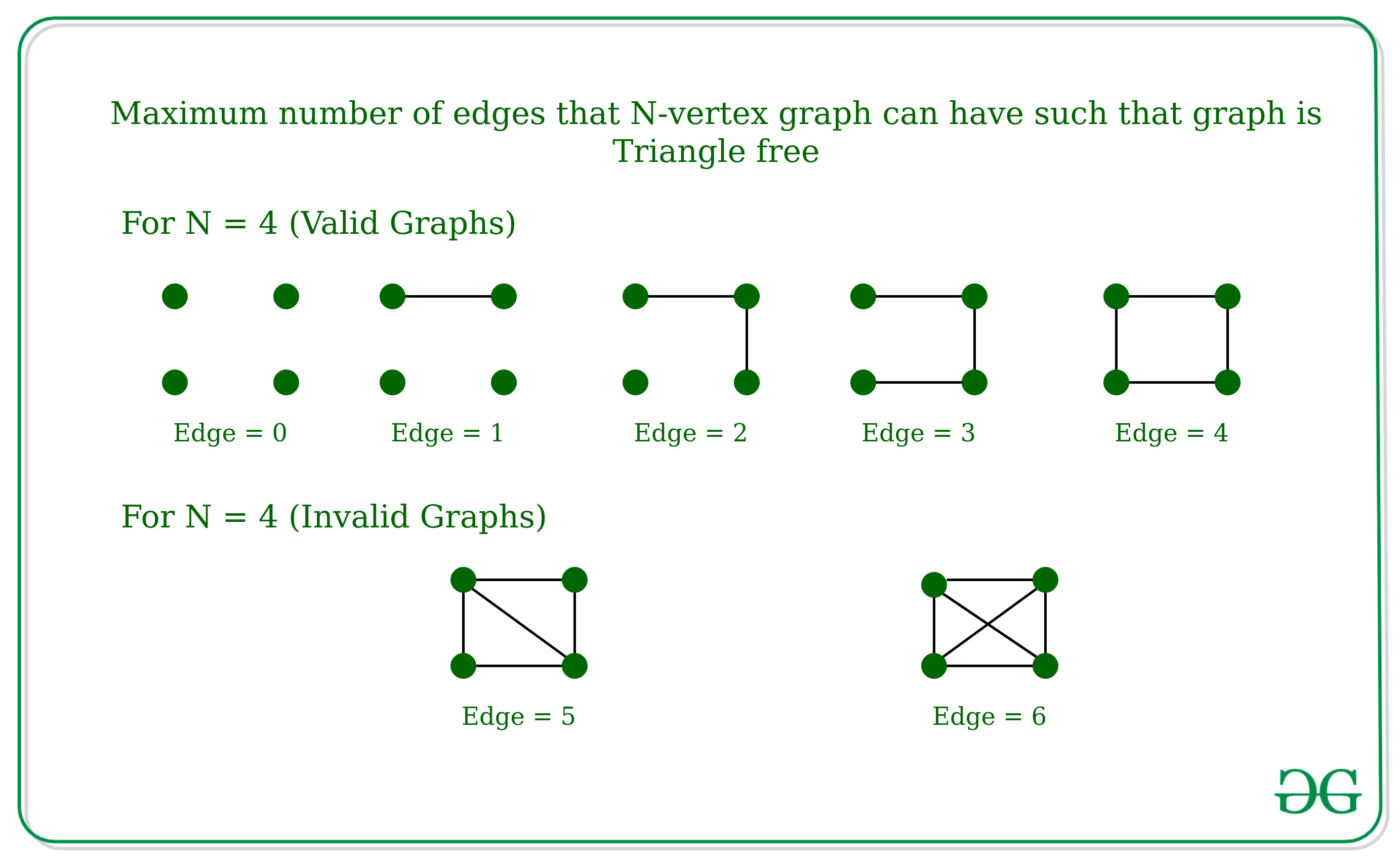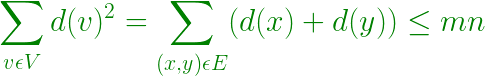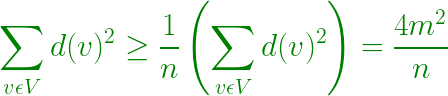# Maximum number of edges that N-vertex graph can have such that graph is Triangle free | Mantel’s Theorem

• Last Updated : 23 Apr, 2021

Given a number N which is the number of nodes in a graph, the task is to find the maximum number of edges that N-vertex graph can have such that graph is triangle-free (which means there should not be any three edges A, B, C in the graph such that A is connected to B, B is connected to C and C is connected to A). The graph cannot contain a self-loop or multi edges.

Examples:

Attention reader! Don’t stop learning now. Get hold of all the important DSA concepts with the DSA Self Paced Course at a student-friendly price and become industry ready.  To complete your preparation from learning a language to DS Algo and many more,  please refer Complete Interview Preparation Course.

In case you wish to attend live classes with experts, please refer DSA Live Classes for Working Professionals and Competitive Programming Live for Students.

Input: N = 4
Output:
Explanation:Input: N = 3
Output:
Explanation:
If there are three edges in 3-vertex graph then it will have a triangle.

Approach: This Problem can be solved using Mantel’s Theorem which states that the maximum number of edges in a graph without containing any triangle is floor(n2/4). In other words, one must delete nearly half of the edges to obtain a triangle-free graph.

How Mantel’s Theorem Works ?
For any Graph, such that the graph is Triangle free then for any vertex Z can only be connected to any of one vertex from x and y, i.e. For any edge connected between x and y, d(x) + d(y) ≤ N, where d(x) and d(y) is the degree of the vertex x and y.

• Then, the Degree of all vertex –• By Cauchy-Schwarz inequality –• Therefore, 4m2 / n ≤ mn, which implies m ≤ n2 / 4

Below is the implementation of above approach:

## C++

 `// C++ implementation to find the maximum` `// number of edges for triangle free graph`   `#include ` `using` `namespace` `std;`   `// Function to find the maximum number of` `// edges in a N-vertex graph.` `int` `solve(``int` `n)` `{` `    ``// According to the Mantel's theorem` `    ``// the maximum number of edges will be` `    ``// floor of [(n^2)/4]` `    ``int` `ans = (n * n / 4);`   `    ``return` `ans;` `}`   `// Driver Function` `int` `main()` `{` `    ``int` `n = 10;` `    ``cout << solve(n) << endl;` `    ``return` `0;` `}`

## Java

 `// Java implementation to find the maximum ` `// number of edges for triangle free graph ` `class` `GFG ` `{ `   `    ``// Function to find the maximum number of ` `    ``// edges in a N-vertex graph. ` `    ``public` `static` `int` `solve(``int` `n) ` `    ``{` `        `  `        ``// According to the Mantel's theorem ` `        ``// the maximum number of edges will be ` `        ``// floor of [(n^2)/4] ` `        ``int` `ans = (n * n / ``4``); `   `        ``return` `ans; ` `    ``} `   `    ``// Driver code` `    ``public` `static` `void` `main(String args[])` `    ``{ ` `        ``int` `n = ``10``; ` `        ``System.out.println(solve(n)); ` `    ``} ` `}`   `// This code is contributed by divyamohan123`

## C#

 `// C# implementation to find the maximum ` `// number of edges for triangle free graph ` `using` `System;`   `class` `GFG ` `{ `   `    ``// Function to find the maximum number of ` `    ``// edges in a N-vertex graph. ` `    ``public` `static` `int` `solve(``int` `n) ` `    ``{` `        `  `        ``// According to the Mantel's theorem ` `        ``// the maximum number of edges will be ` `        ``// floor of [(n^2)/4] ` `        ``int` `ans = (n * n / 4); `   `        ``return` `ans; ` `    ``} `   `    ``// Driver code` `    ``public` `static` `void` `Main()` `    ``{ ` `        ``int` `n = 10; ` `        ``Console.WriteLine(solve(n)); ` `    ``} ` `}`   `// This code is contributed by AnkitRai01`

## Python3

 `# Python3 implementation to find the maximum` `# number of edges for triangle free graph`   `# Function to find the maximum number of` `# edges in a N-vertex graph.` `def` `solve(n):` `    `  `    ``# According to the Mantel's theorem` `    ``# the maximum number of edges will be` `    ``# floor of [(n^2)/4]` `    ``ans ``=` `(n ``*` `n ``/``/` `4``)`   `    ``return` `ans`   `# Driver Function` `if` `__name__ ``=``=` `'__main__'``:` `    ``n ``=` `10` `    ``print``(solve(n))`   `# This code is contributed by mohit kumar 29`

## Javascript

 ``

Output:

`25`

Time Complexity: O(1)

My Personal Notes arrow_drop_up
Recommended Articles
Page :